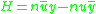xLagrange invariantEncyclopedia
In optics
Optics
Optics is the branch of physics which involves the behavior and properties of light, including its interactions with matter and the construction of instruments that use or detect it. Optics usually describes the behavior of visible, ultraviolet, and infrared light...

the Lagrange invariant is a measure of the light propagating through an optical system. It is defined by,
where y and u are the marginal ray height and angle respectively, and ȳ and ū are the chief ray height and angle. n is the ambient refractive index
Refractive index
In optics the refractive index or index of refraction of a substance or medium is a measure of the speed of light in that medium. It is expressed as a ratio of the speed of light in vacuum relative to that in the considered medium....

. In order to reduce confusion with other quantities, the symbol Ж may be used in place of H. Ж2 is proportional to the throughput of the optical system (related to étendue
Etendue
Etendue or étendue is a property of pencils of rays in an optical system, which characterizes how "spread out" light is in area and angle. It may also be seen as a volume in phase space....

). For a given optical system, the Lagrange invariant is a constant throughout all space, that is, it is invariant upon refraction
Refraction
Refraction is the change in direction of a wave due to a change in its speed. It is essentially a surface phenomenon . The phenomenon is mainly in governance to the law of conservation of energy. The proper explanation would be that due to change of medium, the phase velocity of the wave is changed...

and transfer.

The optical invariant is a generalization of the Lagrange invariant which is formed using the ray
Ray (optics)
In optics, a ray is an idealized narrow beam of light. Rays are used to model the propagation of light through an optical system, by dividing the real light field up into discrete rays that can be computationally propagated through the system by the techniques of ray tracing. This allows even very...

heights and angles of any two rays. For these rays, the optical invariant is a constant throughout all space .